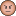# QlikView App Development

Discussion Board for collaboration related to QlikView App Development.Not applicable

## How to calculate the moving difference in a Pivot Table

I have a Pivot table, with Dimension 1 = Customer Region, Dimension 2 = Order Date. It looks like

Region Order Diff Order Diff Order Diff Order Date Jan 1, 2012 Jan 2, 2012 Jan 3, 2012 NY 100 - 80 -20 60 -20 CT 35 - 40 5 20 -20 NJ 22 - 33 11 11 -22

While the Expression for Order is simply =Sum(Orders), I don't know how to write the expression for the Diff column, which is the difference of the sum of the orders between the current date and the previous date.

Can anyone show me an example?

Many thanks!

1 Solution

Accepted SolutionsNot applicable

## Re: How to calculate the moving difference in a Pivot Table

You can use Before, to operate in pivot tables, is like Above in Straight Tables.

Try this:  sum(Orders)-Before(sum(Orders))

Hope it helps

6 Replies
Valued Contributor II

## Re: How to calculate the moving difference in a Pivot Table

Try this:

sum( {<[Order Date]={"=[Order Date]"},[Order Date]=>}Orders) - sum( {<[Order Date]={"=[Order Date]-1"},[Order Date]=>}Orders)

may be you want to try each one separately beforeNot applicable

## Re: How to calculate the moving difference in a Pivot Table

Thanks a lot for the help, but this doesn't work as "[Order Date]=" only disregard the filter selection, but the subset is still limited to the current dimension.

Sum(TOTAL<[Customer Region]> Orders) can get the subset that's limited only by the Region, but then {<[Order Date]={"=[Order Date]-1"},[Order Date]=>} isn't doing what I want it to do.Valued Contributor III

## Re: How to calculate the moving difference in a Pivot Table

Please see if the attached solution will be acceptable.Not applicable

## Re: How to calculate the moving difference in a Pivot Table

You can use Before, to operate in pivot tables, is like Above in Straight Tables.

Try this:  sum(Orders)-Before(sum(Orders))

Hope it helpsNot applicable

## Re: How to calculate the moving difference in a Pivot Table

Thanks a lot for the help. I wasn't aware of the Before() function.

Contributor

## Re: How to calculate the moving difference in a Pivot TableI'm looking for something similar but with above result. The difference column needs to a column of its own. Suggestions please?